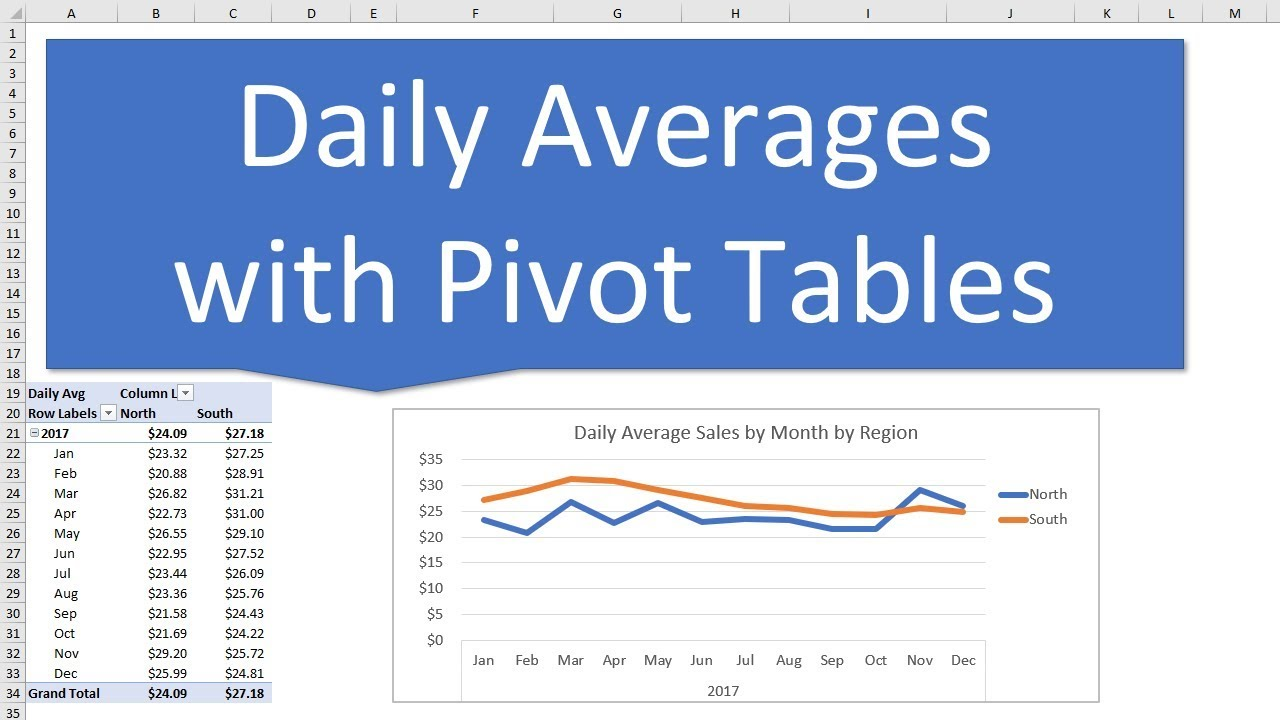How to Calculate Total Daily Averages with Pivot TablesLearn how to calculate the average of a daily total amount across month, quarters, years, or even categories with a pivot table.
Learn how to calculate the average of a daily total amount across month, quarters, years, or even categories with a pivot table.

In this example the source data contains data for a sales transaction on each row. There are multiple sales per day, and therefore multiple rows with the same date.

This daily average calculation allows us to sum up multiple rows of data for the same date, and find the average trend across weeks, months, quarters, years, etc.

For this technique we will use the Power Pivot Data Model and DAX Measures. Don't let that scare you, as I explain it all step-by-step.

IMPORTANT: You will need Excel 2013 or later for Windows. That includes an Office 365 subscription. I will post another video in the future on how to do this on Excel 2010, or Excel for Mac.

Here are the 3 measures we create to calculate the Average Daily Sales:

1. Total Sales = SUM([Amount])
2. Distinct Day Count = DISTINCTCOUNT([Date])
3. Daily Average = [Total Sales]/[Distinct Day Count]

The DISTINCTCOUNT function in DAX calculates the distinct count of days for any given filter context in the pivot table. We take Total Sales for the same intersection and divide it by Distinct Count of days (date column) to get the Daily Average.

The great part about this technique is that it works for any time period grouping or even comparison between categories like region, department, etc.